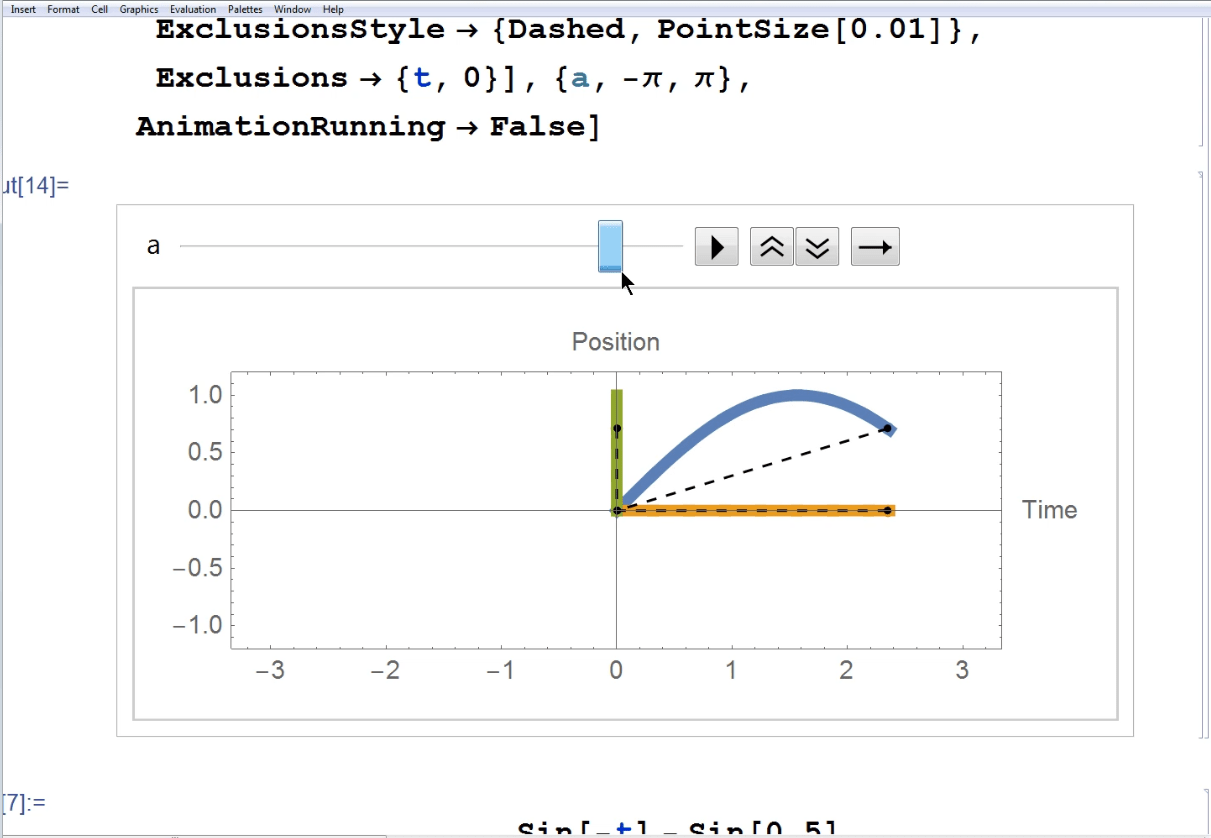4
Sep 17

# Geometry related to derivatives

In a sequence of videos I explain the main ideas pursued by the fathers of Calculus - Isaac Newton and Gottfried Wilhelm Leibniz.

## Derivative equals speed

Here we assume that a point moves along a straight line and try to find its speed as usual. We divide the distance traveled by the time it takes to travel it. The ratio is an average speed over a time interval. As we reduce the length of the time interval, we get a better and better approximation to the exact (instantaneous) speed at a point in time.

## Position of point as a function of time

Working with the visualization of the point movement on a straight line is inconvenient because it is difficult to correlate the point position to time. It is much better to visualize the movement on the space-time plane where the horizontal axis is for time and the vertical axis is for the point position.

##Video 2. Position of point as function of time

## Measuring the slope of a straight line

A little digression: how do you measure the slope of a straight line, if you know the values of the function at different points?

## Derivative as the slope of a tangent line

This is like putting two and two together: we apply the previous definition to the slope of a secant drawn through two points on a graph. Then it remains to notice that the secant approaches the tangent line, as the second point approaches the first.

## From function to its derivative

This is a very useful exercise that allows later to come up with the optimization conditions, called first order and second order conditions.

## Conclusion

Let$P(t)$ be some function and fix an initial point$t_1$. The derivative$P^\prime(t_1)$ is defined as the limit$P^\prime(t_1)=\lim_{t_2\rightarrow t_1}\frac{P(t_2)-P(t_1)}{t_2-t_1}.$

When$P(t)$ describes the movement of a point along a straight line, the derivative gives the speed of that point. When$P(t)$ is drawn on a plane, the derivative gives the slope of the tangent line to the graph.